Mobile QR Code1. (Department of Electronic Engineering, Myongji University, Yongin 17058, Korea )
2. (Department of Electrical and Computer Engineering, Ajou University, Suwon 16499, Korea)

Tunneling field-effect transistor (TFET), line tunneling FET (LTFET), partial SiGe source, partial light doping source, subthreshold swing (SS), on-off current ratio (ION/IOFF)

## I. INTRODUCTION

While metal oxide-semiconductor field-effect transistor (MOSFET) cannot achieve a subthreshold swing (SS) below 60 mV/dec at room temperature due to the limitation by thermionic emission, tunneling field-effect transistor (TFET) is allowed to have SS of sub-60-mV/dec by its injection mechanism of band-to-band tunneling (BTBT) . On the other hand, TFET has a weakness of low current drivability, which has called for researches on various materials and novel structures to overcome the weakness and achieve a smaller SS. One of the novel structures proposed to improve the electrical characteristics of TFETs is line tunneling field-effect transistor (LTFET) [2,3]. In the LTFET, the electrical characteristics are mainly governed by vertical tunneling in the source-to-gate direction. However, the effect of lateral tunneling in the source-to-drain direction has been seldom reported in detail [4,5]. In this work, the change in lateral tunneling is investigated by adjusting the material and doping concentration at the tip of the source junction.

## II. TUNNELING MODEL CALIBRATION

In order to calibrate the BTBT model using Sentaurus tools, the planar Si and SiGe TFETs which are fabricated are used [Fig. 1(a) and (b)]. The SiGe TFET consists of 40 nm thickness Si$_{\mathrm{0.7}}$Ge$_{\mathrm{0.3}}$ layers on SOI (100) substrates which is lightly p-doped (1 ${\times}$ 10$^{15}$ cm$^{-3}$) with a thickness of 60 nm and Si capping layer grown on the SiGe channel and 200 nm thickness poly-Si gate and 3 nm SiO$_{2}$. In the case of Si TFET, SOI thickness is 100 nm. BF$_{2}$ with dose of 8 ${\times}$ 10$^{14}$ cm$^{-2}$, 7$^{\circ}$ tilt, and 10 keV acceleration energy are used for the source implantation and drain implantation with As is used at the same conditions. . The transfer curves of the fabricated Si and SiGe TFETs with both 400 nm gate length and width are measured at the drain voltage (V$_{\mathrm{DS}}$) of 1.0 V .

##### Fig. 1. Cross-sectional views of the (a) Si TFET; (b) SiGe TFET which is fabricated for calibration. Calibrated transfer curves of (a) Si; (b) SiGe TFET.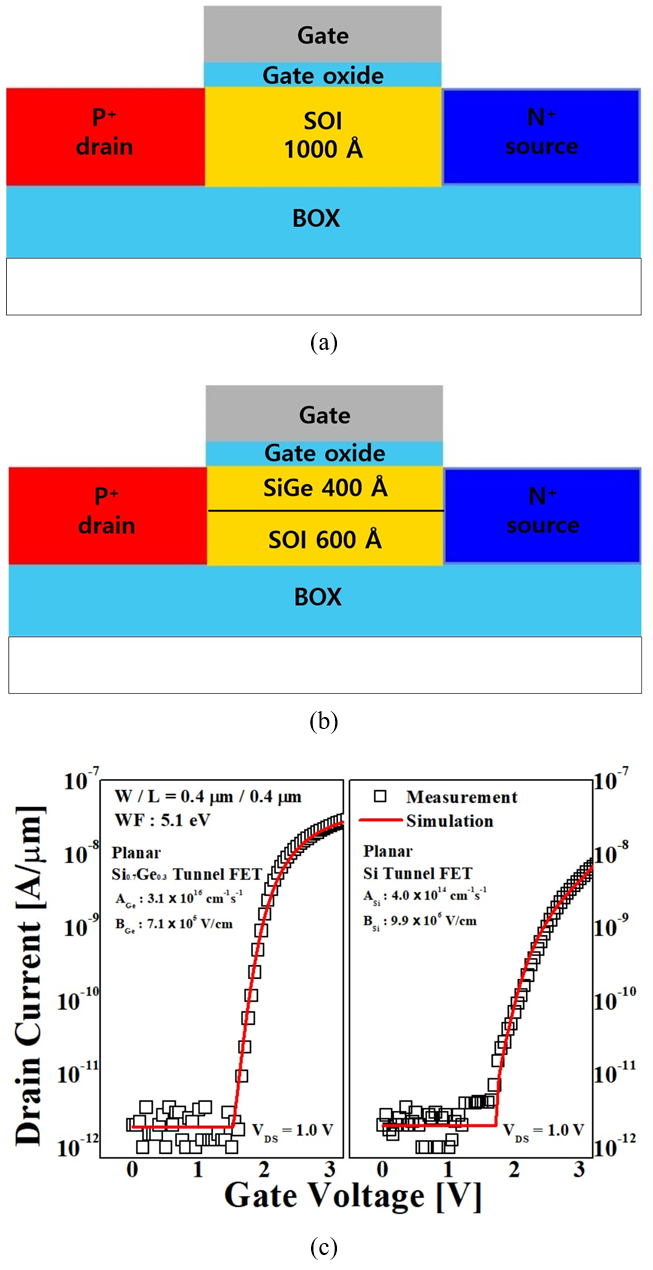Kane’s BTBT model is calibrated to the measured result of the fabricated Si and SiGe TFETs. The BTBT generation rates (G) per unit volume in this model are defined as in the uniform electric field limit where F$_{0}$ = 1 V/cm and P = 2.5 for indirect BTBT . A of the pre-factor and B of the exponential factor are the Kane parameters while F is the electric field [9,10]. As mentioned above, a number of simulations are executed to fit the measured data by adjusting the A and B parameters. The A and B are selected as for optimal fit according to the results between the simulated and measured data [Fig. 1(c)]. It can be seen that transfer curves are well fitted from the result. The extracted A and B parameter of the BTBT model in Si TFET are 4 ${\times}$ 10$^{14}$ cm$^{-1}$·s$^{-1}$ and 9.9 ${\times}$ 10$^{6}$ V/cm, respectively. As SiGe TFET’s transfer characteristics, the A and B parameters of Ge can be extracted as 3.1 ${\times}$ 10$^{16}$ cm$^{-1}$·s$^{-1}$ and 7.1 ${\times}$ 10$^{5}$ V/cm, respectively.

Fig. 2 shows the reference structure of the LTFET which is composed of Ge source, Si body, Si drain and Aluminum gate (4.1 eV work function). Because Ge has valence band offset from Si, using Ge source instead of Si generates more BTBT at the same voltage . Therefore, in this paper, Ge source is basically used for our proposed structure. And the closer the drain is to the gate, the more severe the ambipolar characteristics, so the drain is placed farther from the gate like the source structure.

##### Fig. 2. Reference structure of the LTFET. The length of the drain is also ‘L$_{\mathrm{TUN}}$'. And the gate work function is 4.1 eV.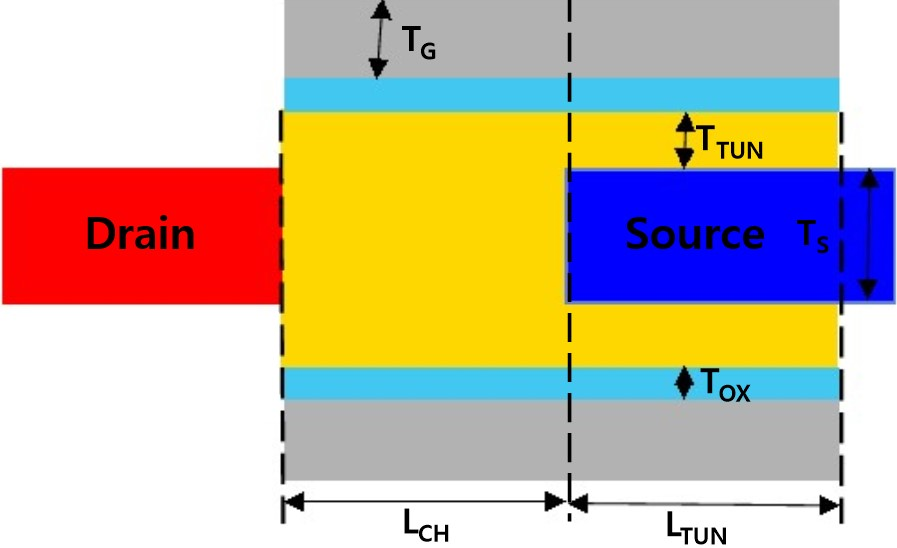##### Table 1. Parameters of the Reference structure used for numerical simulation
 Definitions Value Source doping concentration (Boron) 1020 cm-3 Channel doping concentration (Arsenic) 1017 cm-3 Drain doping concentration (Arsenic) 1020 cm-3 Gate thickness (TG) 10 nm Gate oxide thickness (TOX) 1 nm Channel length (LCH) 30 nm Vertical tunneling length (LTUN) 20 nm Vertical tunneling thickness (TTUN) 2 nm Source and drain thickness (TS) 14 nm Drain Voltage 0.5 V
$\begin{equation*} G=A\left(\frac{F}{F_{0}}\right)^{p}\exp \left(-\frac{B}{F}\right) \end{equation*}$

## III. PARTIALLY LIGHTLY DOPED SOURCE

Despite of using Ge source on LTFET, The on-state current (I$_{\mathrm{ON}}$) is significantly lower than that of MOSFETs due to the limitation of the mechanism called BTBT that generating an electric current, which is different from MOSFETs . For this reason, increasing on-current (I$_{\mathrm{ON}}$) is the most important issue in TFETs. To improve this problem, we propose partially lightly doped source structure [Fig. 3]. By partially reducing doping at the end of the source, a depletion region can be formed at the point where doping concentration decreases at source. Because the depletion region is created within source, the energy band is bent within the source and that make BTBT occurred in Ge unlike the Reference structure in which BTBT occurred in Si [Fig. 4]. Different from Si, Direct BTBT which occurs even at relatively small voltage is dominant in Ge. Therefore, it can be seen that the I$_{\mathrm{ON}}$ increases more than when it is a reference structure [Fig. 5(a)].

As the length of the less doped region is gradually increased, the region where the energy band is bent increases, and the area where BTBT occurs in Ge increases in the source. Thus, when the length of less doped part is long, more direct BTBT is generated than the short one and also its I$_{\mathrm{ON}}$ is bigger than the short one [Fig. 5(b)]. But when the length of the lightly doped region increases beyond a certain level, we can check that the I$_{\mathrm{ON}}$ gradually decreases at 0.7 V or more [Fig. 5(b)]. This is because up to 6 nm length, the effect of increasing Ge region where direct BTBT occurs is large, but from 7 nm, the change of the energy band at the junction becomes gentle and the effect of increasing the width of the tunneling barrier becomes greater [Fig. 5(c)]. Thus, 6 nm is appropriate for the length of partially lightly doping region which has a greater effect of increasing the Ge region where direct BTBT occurs.

##### Fig. 3. Partially lightly doped source structure. Partial light doping is used at the end of the Ge source. Doping concentration (Boron) of partially lightly doped source is 10$^{17}$cm$^{-3}$. L$_{\mathrm{JUNC}}$ is the length of partially lightly doped source.##### Fig. 4. Two-dimensional (2D) plot of BTBT rates at 0.7 V with (a) Reference structure; (b) Partially lightly doped source structure; (c) BTBT generation rate; (d) Energy band of reference structure and partially lightly doped structure at 0.7~V.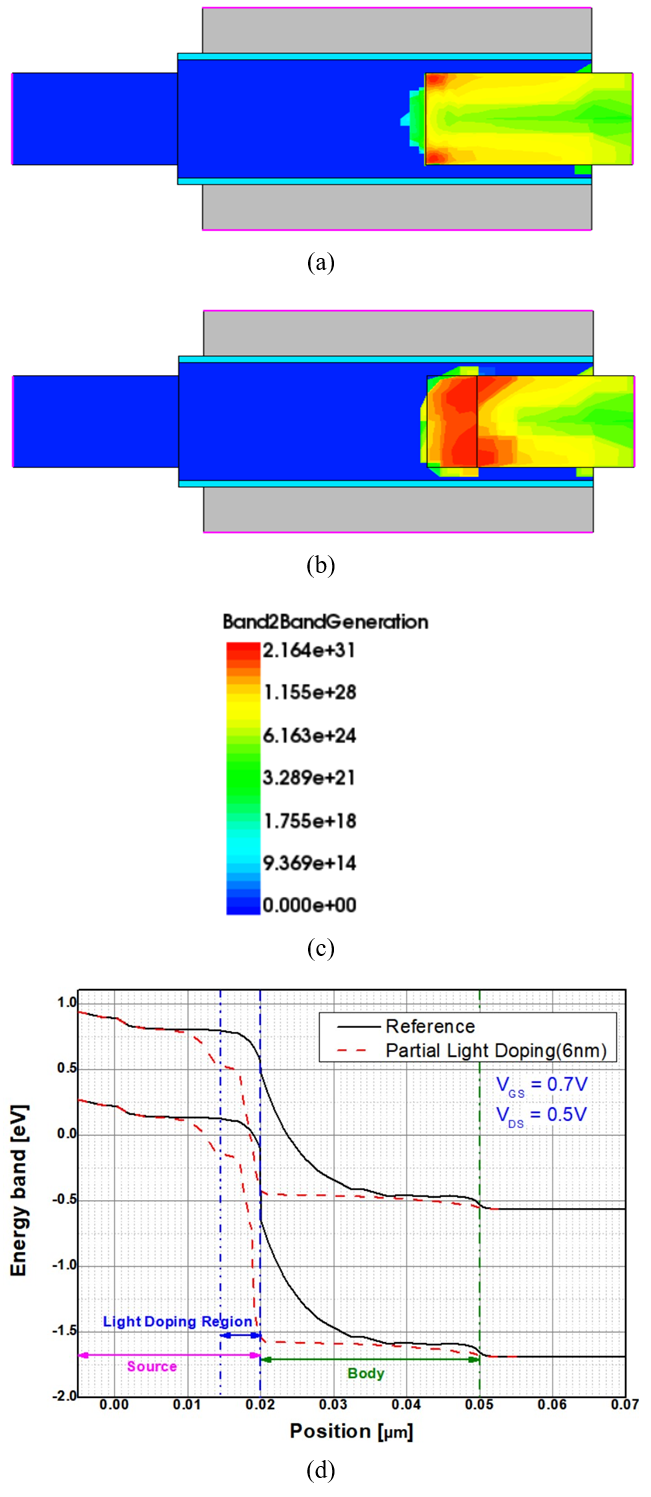##### Fig. 5. (a) Transfer curves of reference structure and partially lightly doped source structure; (b) Transfer curves of partially lightly doped source with different length; (c) Energy band of partially lightly doped source structure with different length at 0.7 V.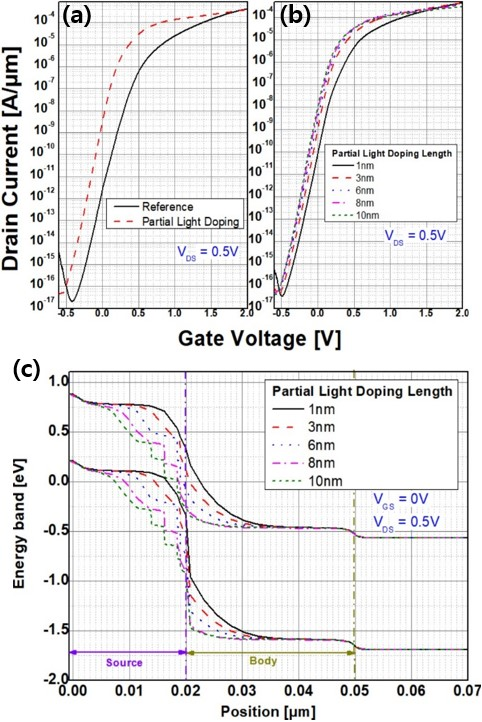## IV. EFFECTS OF PARTIAL SiGe SOURCE

For low power consumption and lower SS, it is necessary for reducing I$_{\mathrm{OFF}}$. In order to reduce I$_{\mathrm{OFF}}$, we use Partial SiGe source at the end of the reference’s source [Fig. 6]. The electric field is mainly focused on the edge part, so point tunneling occurs dominantly at 0~V. If SiGe is partially applied to the end of the Ge source where point tunneling occurs, BTBT is generated less when all Ge source is used due to valence band offset of Ge and SiGe [Fig. 7]. As a result, partial SiGe source structure’s I$_{\mathrm{OFF}}$ is less than that of reference structure [Fig. 8(a)]. The lower Ge content in SiGe, the lower the valence band and the conduction band are similar regardless of the Ge content [Fig. 8(c)]. The lower Ge content in SiGe, the less BTBT occurs at 0 V. Fig. 8(b) shows that the I$_{\mathrm{OFF}}$ is reduced as the Ge content is reduced.

##### Fig. 6. Partial SiGe source structure. SiGe is partially used at the end of Ge source with various Ge content.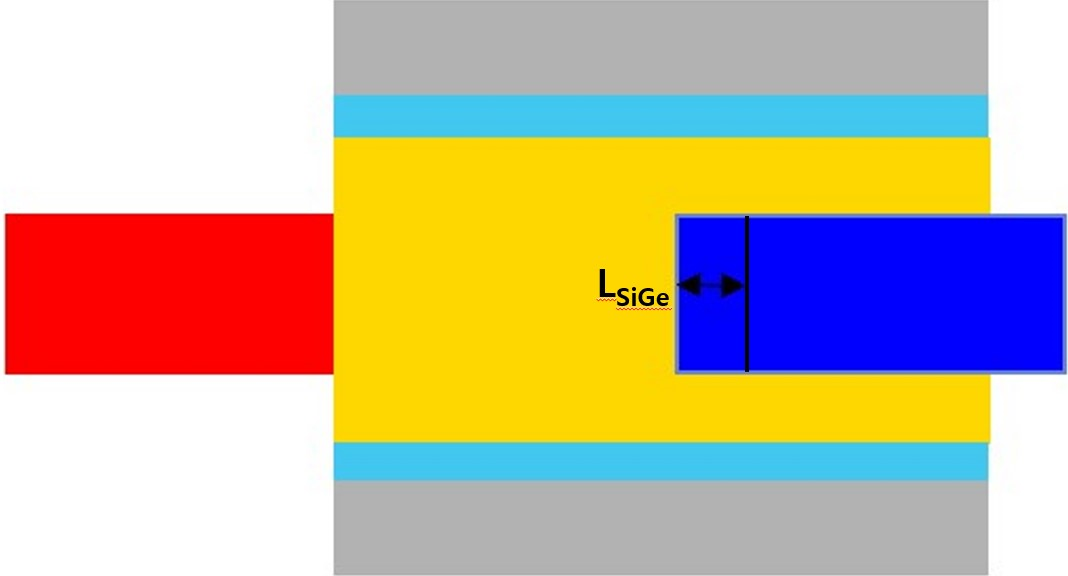##### Fig. 7. Two-dimensional (2D) plot of BTBT rates at 0 V with (a) Reference structure; (b) Partial SiGe source structure with Ge content 10 %; (c) BTBT generation rate. This figure shows that point tunneling decreases when going from reference structure to partial SiGe source structure.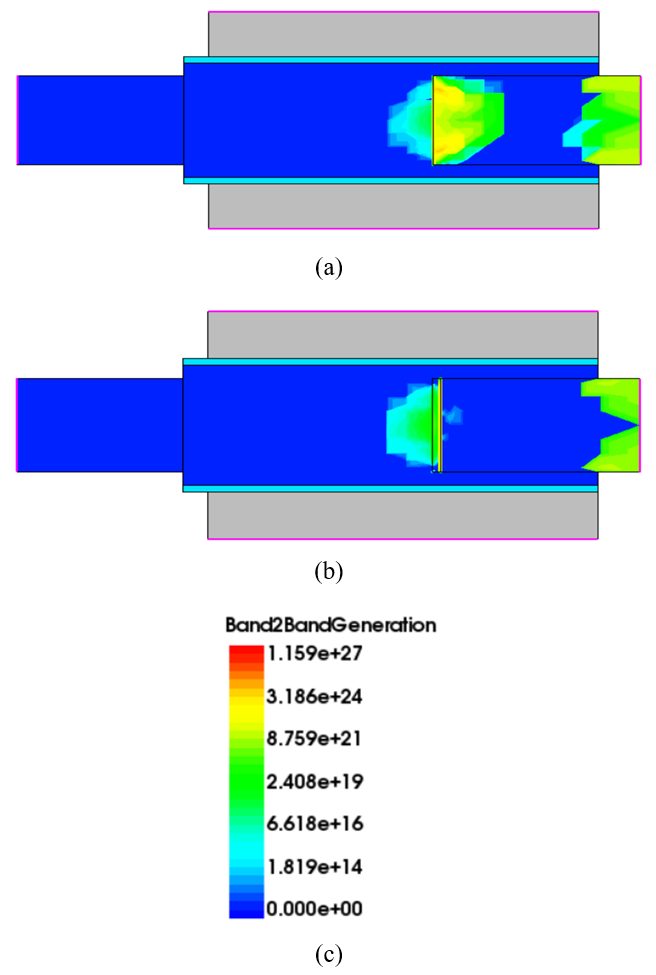##### Fig. 8. (a) Transfer curves of reference structure and partial SiGe source structure; (b) Transfer curves of reference structure and partial SiGe source structure with Ge content 80 % and 10 %; (c) Energy band of reference and partial SiGe source structure with Ge content 80 % and 10 % at 0 V.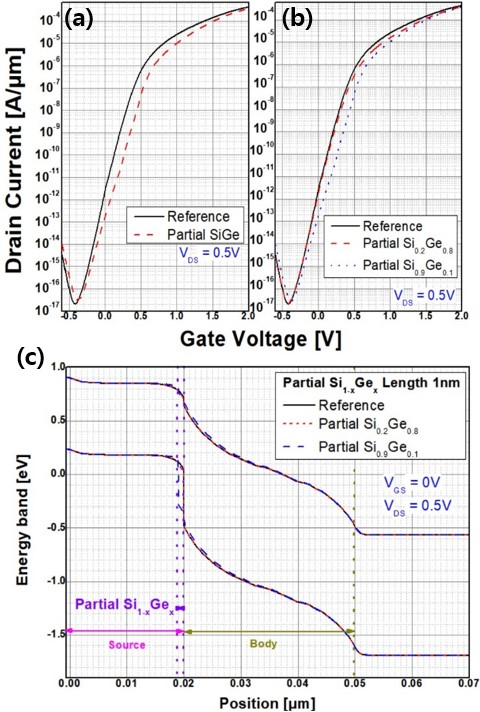## V. EFFECTS OF PARTIALLY LIGHTLY DOPED SOURCE WITH PARTIAL SiGe

In lightly doped source structure, light doping causes BTBT in the Ge region as the energy band bends within the source even at 0 V [Fig. 10]. So this proposed structure has higher I$_{\mathrm{OFF}}$ than reference structure [Fig. 5(a)]. In partial SiGe structure, partial SiGe reduce BTBT rates than that of reference structure even at 0.7 V [Fig. 11]. So this proposed structure has lower I$_{\mathrm{ON}}$ than reference structure [Fig. 8(a)].

To solve each proposed structure’s disadvantage, we propose a structure that puts these two structure together [Fig. 9]. This structure has higher I$_{\mathrm{ON}}$ than reference structure because of partially lightly doped source and lower I$_{\mathrm{O}}$$_{\mathrm{FF}}$ than partially lightly doped source structure because of partial SiGe source [Fig. 12]. This proposed structure has a better on/off ratio than when only lightly doped source is used. Because the energy band is more bent in the on state when a gate voltage of 0.7 V is applied than in off state when a gate voltage of 0 V is applied, the BTBT proportion of the Ge region where direct BTBT occurs within the source becomes large at 0.7 V than at 0 V [Fig. 13(a) and (b)]. Due to this, the I$_{\mathrm{ON}}$ is maintained similarly regardless of the mole fraction of Ge in SiGe region, and in the off state of 0 V, the influence of the Ge region is relatively small, and as the mole fraction of Ge decreases in the SiGe region, the I$_{\mathrm{OFF}}$ also decreases. Thus, as the Ge mole fraction decreases in SiGe region, the I$_{\mathrm{OFF}}$ decreases more than the I$_{\mathrm{ON}}$ decreases [Fig. 13(c)]. As a result of the on/off ratio calculations, when Ge mole fraction is 0.1, the on/off ratio is about 2.1 times higher than when Ge mole fraction is 1 and about 1.25 times higher than when Ge mole fraction is 0.8. Therefore, it is considered good to take the Ge mole fraction to 0.1, reducing the I$_{\mathrm{OFF}}$ and bringing the I$_{\mathrm{ON}}$ similar.

As the longer the partial SiGe region within the partially lightly doped source region, the I$_{\mathrm{OFF}}$ is reduced and the on/off ratio is become better for the same reason as above [Fig. 13(d)]. However, when the partial SiGe region is longer than the partially lightly doped region, the I$_{\mathrm{ON}}$ mainly occurs in the SiGe part, not the Ge part. So the low I$_{\mathrm{ON}}$ problem which is the biggest troubble of TFET cannot be solved. Thus, it is better to have a small SiGe area for high I$_{\mathrm{ON}}$.

##### Fig. 9. Partially lightly doped source with partial SiGe source structure. Light doping is also applied at partial SiGe region.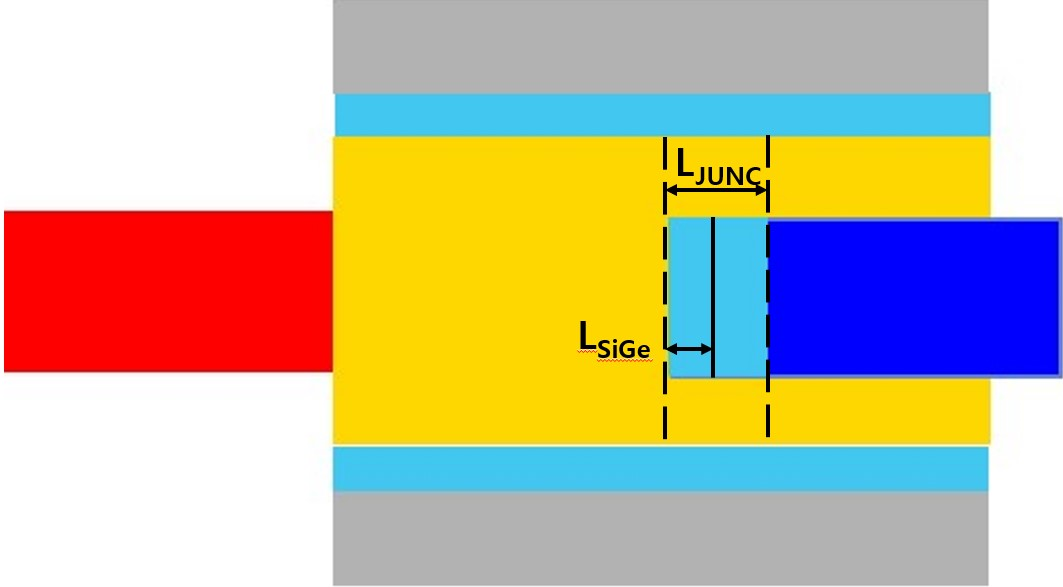##### Fig. 10. (a) Energy band of reference structure and partially lightly doped source structure at 0 V. Two-dimensional (2D) plot of BTBT rates at 0 V with (b) Reference structure; (c) Partially lightly doped source structure; (d) BTBT generation rates.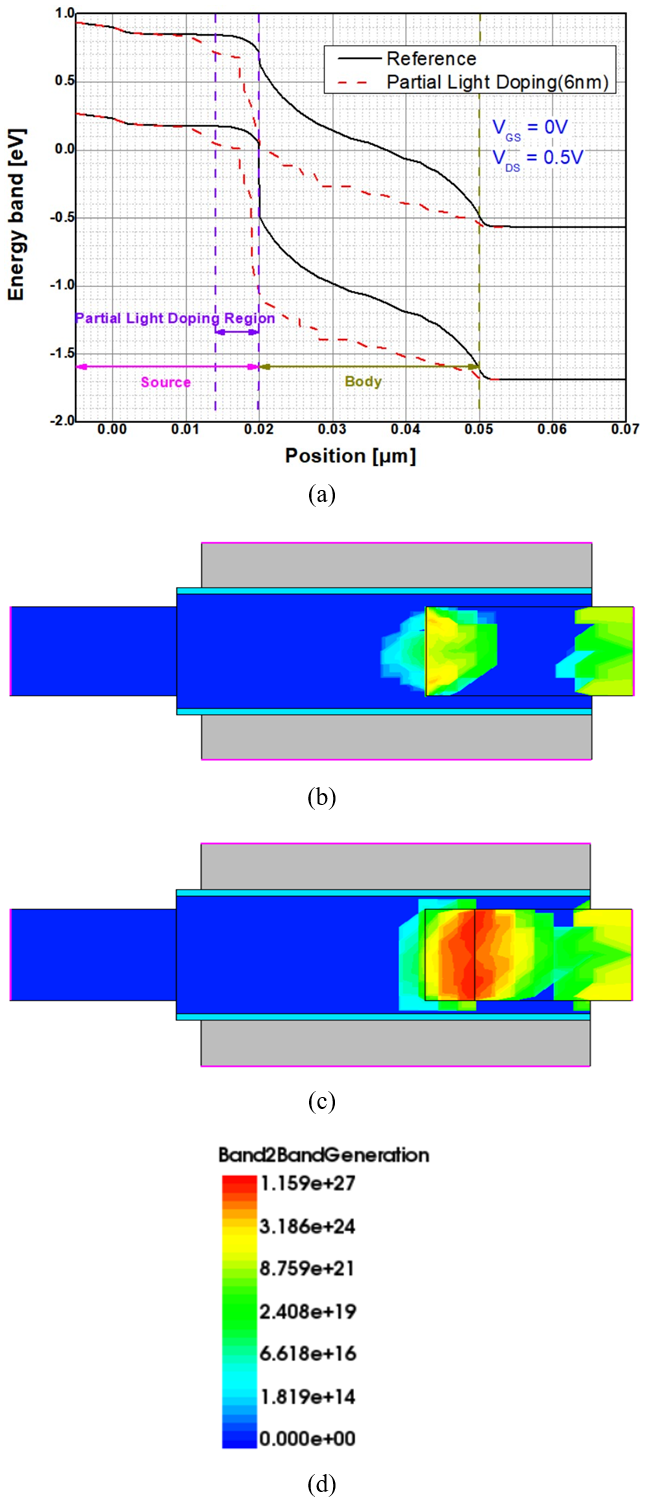##### Fig. 11. Two-dimensional (2D) plot of BTBT rates at 0.7V with (a) Reference structure; (b) Partial SiGe source structure with Ge content 10 %; (c) BTBT generation rate.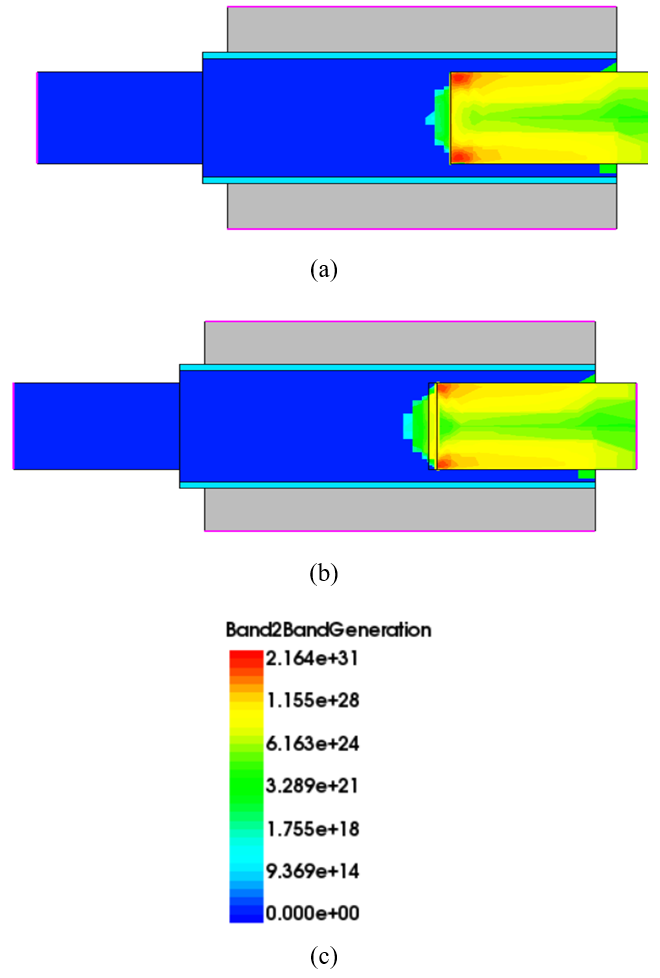##### Fig. 12. Transfer curves of reference structure, partially lightly doped source structure, partial SiGe source structure and partially lightly doped source with partial SiGe source structure.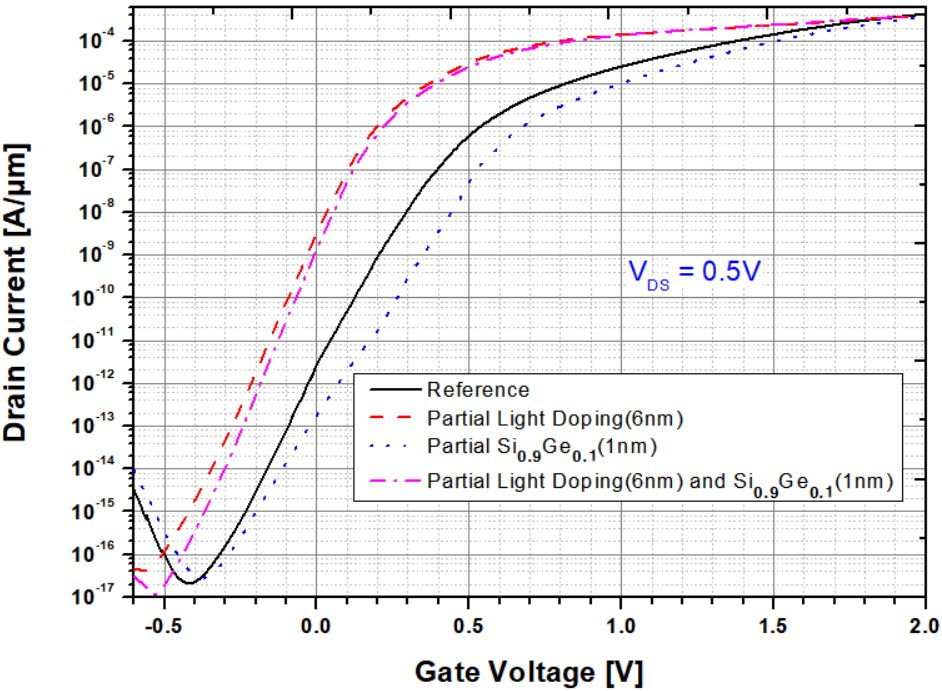##### Fig. 13. Energy band of partially lightly doped source with partial SiGe source structure: (a) at 0 V; (b) at 0.7 V; (c) Transfer curves of partially lightly doped source structure and with Ge content 80 % and 10 % of partial SiGe; (d) Transfer curves of partially lightly doped source and with Ge mole fraction 0.1 of partial SiGe with different Partial SiGe length.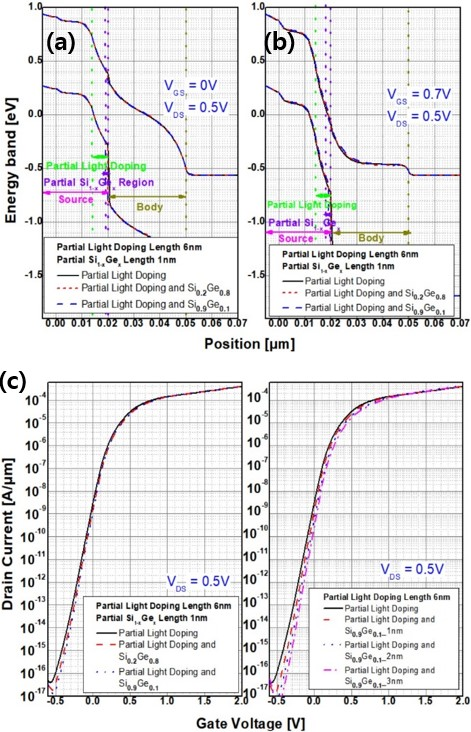## VI. CONCLUSION

In this work, it has been confirmed that the performances of LTFET can be improved by adjusting the material and doping profile at the end of the source junction. Lowering the doping concentration in the source edge leads to higher I$_{\mathrm{ON}}$. A Partial introduction of SiGe at the end of Ge source demonstrates has an effect of reducing I$_{\mathrm{OFF}}$. But Partial Light doping source structure has high I$_{\mathrm{OFF}}$ and Partial SiGe source structure has low I$_{\mathrm{ON}}$. By combining these two structures, I$_{\mathrm{ON}}$ is similar to the partial light doping source structure, but I$_{\mathrm{OFF}}$ is further reduced. And the on/off ratio of optimized structure is improved about twice compared to the lightly doped source structure. This approach make both low-power and high-performance designs viable.

## ACKNOWLEDGMENTS

This work was supported by the National Research Foundation of Korea (NRF) grant funded by the Korea government. (MSIT) (2022R1A2C1093201) The EDA tool was supported by the IC Design Education Center (IDEC), Korea.

## References

1
S. Cho, I. M. Kang, T. I. Kamins, B.-G. Park, and J. S. Harris: Silicon-compatible compound semicon-ductor tunneling field-effect transistor for high performance and low standby power operation, Appl. Phys. Lett., vol. 99, p. 243505, 2011.2
A. M. Walke, et al.: Fabrication and Analysis of a Si/Si0.55Ge0.45 Heterojunction Line Tunnel FET, IEEE Trans. Electron Devices, vol. 61, p. 707, 2014.3
Y. S. Kwon, S. -H. Lee, Y. Kim, G. Kim, J. H. Kim, S. Kim: Surrounding Channel Nanowire Tunnel Field-Effect Transistor with Dual Gate to Reduce a Hump Phenomenon, J. Nanosci. Nanotechnol., vol. 20, p. 4182, 2020.4
W. Vandenberghe, et. al: Analytical model for point and line tunneling in a tunnel field-effect transistor, SISPAD 2008, pp. 137-140.5
Hyun woo Kim, Daewoong Kwon: Low-Power Vertical Tunnel Field-Effect Transistor Ternary Inverter, ElECTRON DEVICES SOCIETY, pp. 286-293, 20216
7
Jang Hyun Kim, Hyun Woo Kim, Seong-Su Shin, Sangwan Kim, and Byung-Gook Park: Transient Analysis of Tunnel Field-Effect Transistor with Raised Drain, Journal of Nonoscience and Nanotechnology, vol. 19, 6212-6216, 2019.8
Sentaurus Device User Guide, ver. G-2012.06, Synopsys Inc.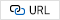9
Kane, E., Theory of tunneling: Journal of Applied Physics, 32(1), p. 83-91, 1961.10
Biswas, A., Dan, S., Royer, C., Grabinski, W. and Ionescu, A., TCAD simulation of SOI TFETs and calibration of non-local banc-to-band tunneling model, Microelectronic Enginnering, 98, p. 334-337, 2012.11
Kuo-Hsing Kao, Student Member, IEEE, Anne S. Verhulst, William G. Vandenberghe, Student Member, IEEE, Bart Sor, Member, IEEE, Guido Groeseneken, Fellow, IEEE, and Kristin De Meyer, Fellow, IEEE: Direct and Indirect Band-to-Band Tunneling in Germanium-Based TFETs, IEEE TRANSACTIONS ON ELECTRON DEVICES, VOL. 59, NO. 2, 2012.12
Gopalakrishnan, K., Griffin, P.B. and Plummer J.D: A Novel Semiconductor Device with a Subthreshold Slope Lower than kT/q. IEEE International Electron Device Meeting (IEDM), pp. 289-292, 2002.Min-Ki Ko is pursing the B.S. degree in the Department of Electronic Engineering from Myongji University, Yongin. His current research interests include tunnel FETs, neuromorphic devices, and capacitor-less 1T DRAMs

Jang Hyun Kim completed his Bachelor's degree in Electrical and Electronic Engineering at KAIST (Korea Advanced Institute of Science and Technology) from March 2005 to August 2009. He then pursued his Master's degree in Dept. of Electrical and Computer Engineering at Seoul National University, from September 2009 to August 2011. Continuing his academic journey, he obtained his Doctorate degree in Dept. of Electrical and Computer Engineering. After completing his education, Jang Hyun Kim worked as a Development Researcher for DRAM (Dynamic Random-Access Memory) at SK hynix from September 2016 to February 2020. Subsequently, he served as an Assistant Professor in the Department of Electrical Engineering at Pukyong National University from March 2020 to February 2023. Currently, he holds the position of Assistant Professor in the Department of Electrical and Computer Engineering at Ajou University, starting from February 2023. His current research interests include logic semiconductor devices and power semiconductor devices.

Garam Kim received the B. S. and the Ph.D. degrees in electrical engineering from Seoul National University, Seoul, Korea, in 2008 and 2014, respectively. He worked as a senior engineer at Samsung Electronics from 2014 to 2019. In 2019, he joined the Department of Electronic Engineering at Myongji University, Yongin, where he is currently an assistant professor. His current research interests include GaN-based LEDs, tunnel FETs, neuromorphic devices, capacitor-less 1T DRAMs, nagative capacitance FETs, FinFETs, and CMOS image sensors.